## Recommended Posts

There is a sign convention with $F=-\frac{GMm}{r^2}$ and subsequenty $U=-\frac{GMm}{r}$ The negative sign in this case indicates gravity as being a downward force towards the centre of mass. Many books however typically drop the minus sign but the convention includes it.

Edited by Mordred

##### Share on other sites

When negative mass and positive mass co-exist, gravitational potential energy-2

1. In the presence of negative mass and positive mass, there are several characteristics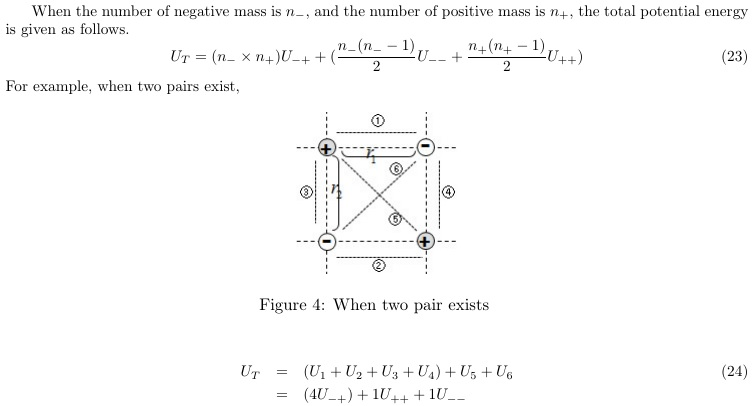1)2 X 2

U_T = (U_1 + U_2 + U_3 + U_4) + (U_5) + (U_6)

U_T = 4(U-+) + 1(U++) + 1(U- -)

2)3 X 3

U_T = 9(U-+) + 3(U++) + 3(U- -)

Gravitational Potential Energy (GPE) shows important characteristics when negative mass and positive mass both co-exist. While n^2 positive (repulsive) gravitational potential terms are produced, n^2 - n negative (attractive) gravitational potential terms are also produced.

The difference in the number of repulsive and attractive terms suggests that there is a difference in the magnitude of the repulsive and the attractive effect.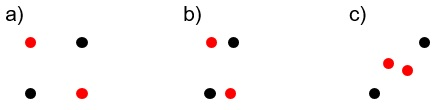Fig.x. Red dot=positive mass, Black dot=negative mass, The U++, U--, and U-+ values vary depending on the arrangement of negative mass and positive mass.

You can get a sense by placing 2X2 particles and doing the direct calculation.

2. Numerical calculations

To look into the characteristic of gravitational potential energy, I used the simulation program named “Gravitation3D” made by Roice Nelson and “G3D File Creator and Reader” made by our team.

A program that can easily simulate the motion characteristics of negative mass :  http://www.gravitation3d.com/

1) Simulation setting

a)Definition of parameter : A few parameters were needed to be defined for simulation.

Distance between pair creation negative energy and positive energy(distance of 1 pair) : d_0,

Minimum distance between particle pairs for density modification during pair creation : d_m,

Radius of pair creation range : R_0 =500,

Particle number of pair creation : N_0 =2,000ea (1,000 pairs)

b)Finding mean value, 1,000 particle pairs (total 2,000ea particles) were produced by random and one mean value (gravitational potential energy) of each distance value was found 5 times each.

c)Verification on program : To check if the calculated results of the program were correct, I calculated the GPE when 1, 2, and 3 pairs (consist of 15ea potentials) of particles existed by hand and confirmed that this value corresponded to the calculated results of the program.

2) Simulation results

a) Distance of 1 pair (d_0) = 0.01, 0.10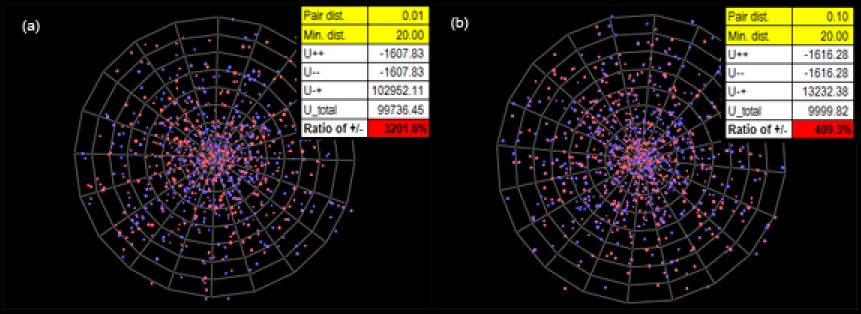(*Please look at the table above and think about the meaning of it.)

It was found out that U-+ value having positive value could be much higher than |U++| + |U- -|. Thus, even though the size of positive mass and negative mass was equal, it could be known that repulsive GPE could be much higher than attractive GPE.

b) Distance of 1 pair (d_0) = 0.19, 1.00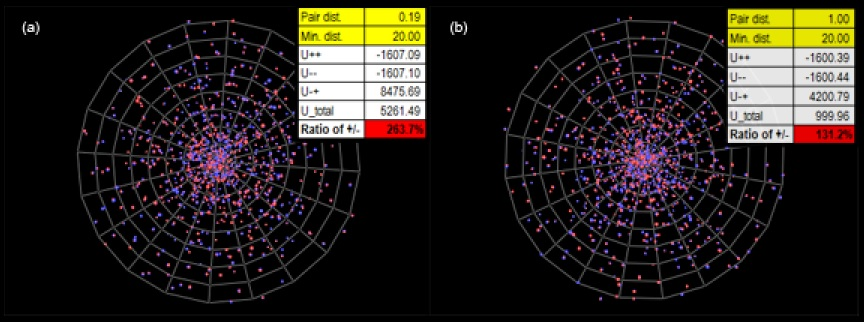Dark matter and matter correspond to negative gravitational potential and dark energy correspond to positive gravitational potential because it produces repulsive effect. Therefore, observation ratio of current universe is 72.1/27.9 = 2.584 (WMAP reference). This shows similar value to 2.63 which was found above. If conditions change, ratio of negative gravitational potential and positive gravitational potential can have various values close to 2.58.

The fundamental ideas or inferences of current mainstream physics and astronomy

"If there are some gravitational effects, there are some gravitational source corresponding to each one of those gravitational effects." And "if the magnitudes of any gravitational effects are a> b> c, then the magnitudes of the energy density (which the source of this gravitational effect,) are ρ_a> ρ_b> ρ_c. "

However, this inference cannot always be established.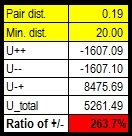U++ = -1607.09

U -- = -1607.10

U-+ = + 8475.69

U-+ is about 5.3 times of |U++|, and it is an anti-gravity term because it is a positive value. In computer simulation, this universe actually expands.

See the GPE U++, U- -, U-+ values in the table above. U-+ has a value about 5.3 times greater than U++ (matter) and is a repulsive GPE term. Let us imagine that we observe this phenomenon under conditions where we do not know the source of gravity. We will assume that there is a repulsive energy density (ρ-+) that is 5.3 times greater than the energy density (ρ++), the origin of U++.

We can see through the above computer simulation that U-+ values with repulsive gravitational effects are much larger than |U--| + |U++|. In other words, even though the magnitudes of the positive and negative masses are the same, the repulsive gravity effect produced by them is much larger than the attractive gravity effect. It is a characteristic that cannot be derived from the model that analyzes the motion with only the total mass in the shell.

By the way, what are the sources of these gravitational potential energy terms U++, U- -, U-+ ?

It is the mass energy of positive and negative masses Σ+mc^2 and Σ-mc^2. Under normal circumstances, the mass energy is much larger than the gravitational potential energy.

In short, three gravitational effects can come from two gravitational sources.

This discovery suggests that mainstream inference (If there are some gravitational effects, there are some gravitational source corresponding to each one of those gravitational effects. And, if the magnitudes of any gravitational effect are a> b> c, then the magnitudes of the energy density (which the sources of this gravitational effects,) are ρ_a> ρ_b> ρ_c. ) do not always hold.

In the field of astronomy, the results of key reasoning and core reasoning may collapse.

And I think it is connected to the dark matter, the dark energy problem.

c) WMAP's result

As a matter of fact, through numerical calculation using a computer, the distribution having a similar value to the predicted rate of WMAP was revealed.

In the Negative Mass Universe Model (Pair Creation Universe Model), negative masses are clustered outside positive mass galaxy or cluster of positive mass galaxies. Therefore, I have calculated the gravitational potential energy in such a batch.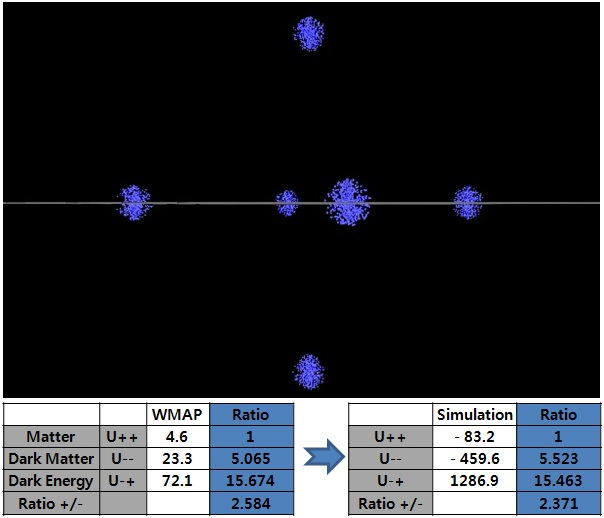Fig.-x. m_+ = +100 X 6 = + 600. (±1200,0,0), (0,±1200,0), (0,0,±1200), each 100. -m_- = (- 0.2 X 500) X 6 = - 600.

Negative mass distribution : center1,2(±1200,0,0), center3,4(0,±1200,0), center5,6(0,0,±1200), negative mass is generated randomly within R=3 ~120, min. distance = 8.

Through the distribution of a negative mass and a positive mass, we could obtain a similar result to WMAP observation or predicted Ratio. This suggests that the currently predicted energy ratio comes from the distribution that negative masses are surrounding the galaxy or the galaxy clusters.

Matter = U++ = - 83.2 ( ratio: 1)

Dark Matter = U- - = - 459.6 ( ratio: 5.523)

Dark Energy = U-+ = + 1286.9 (ratio : +15.463) : repulsive gravitational effect

It is similar the ratio of matter(4.6% : 1) : dark matter(23.3% : 5.06) : dark energy(72.1% : +15.67 : repulsive gravitational effect).

Through the distribution of a negative mass and a positive mass, we could obtain a similar result to WMAP observation or predicted ratio.

Edited by icarus2

##### Share on other sites
12 minutes ago, icarus2 said:

When negative mass and positive mass co-exist, gravitational potential energy-2

1. In the presence of negative mass and positive mass, there are several characteristics

1)2 X 2

U_T = (U_1 + U_2 + U_3 + U_4) + (U_5) + (U_6)

U_T = 4(U-+) + 1(U++) + 1(U- -)

2)3 X 3

U_T = 9(U-+) + 3(U++) + 3(U- -)

Gravitational Potential Energy (GPE) shows important characteristics when negative mass and positive mass both co-exist. While n^2 positive (repulsive) gravitational potential terms are produced, n^2 - n negative (attractive) gravitational potential terms are also produced.

The difference in the number of repulsive and attractive terms suggests that there is a difference in the magnitude of the repulsive and the attractive effect.

Fig.x. Red dot=positive mass, Black dot=negative mass, The U++, U--, and U-+ values vary depending on the arrangement of negative mass and positive mass.

You can get a sense by placing 2X2 particles and doing the direct calculation.

This is reminiscent of the caculation of Madelung constants.

Have you heard of these?

You should be able to derive similar overall constants for your hypothesis.

Now I have done you the courtesy of offering constructive comments and asking a couple of simple questions that can support simple answers rather than long screeds that seem to be prefaced by such get out clauses as " We assume that".........

Now please provide a simple direct answer to a simple direct question.

##### Share on other sites
23 hours ago, icarus2 said:

In short, the current mainstream model is a model based on one kind of gravitational source(positive  mass or energy; mainstream physics describes matter, radiation, dark matter as objects with a positive energy density). By the way, if there are two kinds of gravitational sources, we have to establish new equations.

In addition, some of the existing core reasoning may not be established.

I think and insist on the following.

1) The source of dark matter and dark energy is the negative mass.

2) In the galaxy scale, the dark matter effect (an additional centripetal force effect) is the centripetal force effect produced by the negative mass existing outside the galaxy.

3) The accelerating expansion of the universe is an antigravity effect by negative mass.

4) In the cosmology, dark matter and dark energy correspond to U-- and U-+ terms, respectively. The negative and positive masses have different kinetic characteristics, so the U- - and U-+ values change differently.

5) The expansion of the universe must reflect the change in total gravitational potential energy.

1. Implications from the Friedmann equations

Friedman equation can be induced from 00 component of field equation. But we can also induce this from conservation of energy in classical mechanics, which helps capture the situation definitely.

The dark energy term can be explained by adding a form of potential energy term to the mechanical energy conservation equation.

These are the contents of this book.( Bradley W. Carroll, Dale A. Ostlie. Introduction to Modern Astrophysics.)

We do not know what the sources of dark matter and dark energy are. However, we know that "assuming or introducing something can explain their terms."

The above derivation suggests that, in addition to the potential energy of a material, one attraction term and one repulsion term can explain dark matter and dark energy. Therefore, we should study the properties of gravitational potential energy in the presence of negative mass and positive mass.

2. When negative mass and positive mass co-exist, gravitational potential energy

There are other problems here than in the case of positive mass.

For example, analysis by total mass, which was established in existing positive mass models, is not appropriate or requires extreme caution. When M and -M are uniformly distributed in an arbitrary radius R, the gravity acting on the outside of the system by a total mass in this radius is described as zero. It is possible to arrange the particles so as to have the same size as the center of gravity in terms of the total mass without overlapping the positions of the particles. So there is no change in this analysis.

However, when negative mass and positive mass exist together, the situation is changed because of the existence of U-+ term of gravitational potential energy. There is a kind of internal energy, positive gravitational potential energy. Expansion occur even if the total energy is zero.

Also, the movements of the positive mass and negative mass, the negative and negative mass, and the negative mass and positive mass are different. There are also other heterogeneous situations that are different from when only positive mass exists.

I think that to get accurate results in cosmology, we should consider the total gravitational potential energy rather than a single potential energy such as GMm / r.

Since the distribution of negative mass and positive mass varies and the location of all galaxies changes, the expansion of the universe should reflect the change in total gravitational potential energy.

Gravitational potential energy in the presence of negative mass and positive mass

Now consider U- - term. In cosmology, let us suppose that we do not know what the source of this term is. And we are thinking of objects with a positive mass as a component of the universe.

How do we interpret this term when we observe a negative gravitational potential energy term such as the term U- - , without knowing the source of the gravitational potential energy term? Removing the symbol removes this form –Gmm/r.

Is this interpreted as -8πGρ/3 ? Or interpret it like +8πGρ/3?

In other words, in cosmology based on existing positive mass, the negative gravitational potential energy term U- - seems likely to appear in the Friedmann equation in the form of -8πGρ/3 shape.

There are more complicated things, but anyway, back to the logic of this model, I assert that

The present cosmological constant can be obtained by adding potential U_Λ = - (1/6)Λ m(cr)^2 to mechanical energy conservation equation. If we insert "new potential energy term" into mechanical energy conservation equation, we will get a dark matter term and dark energy term. At this time, let's insert the above new gravitational potential energy(When there is a negative mass and a positive mass, the gravitational potential energy term U_T=U++ + U-- + U-+) term into it.

If U++, U- -, U-+ has a ratio(4.9% : 26.8% : 68.3%) between each other, maybe, we will estimate that ratio of energy density such as 4.9% : 26.8% : 68.3% exist.

This model can prove the energy composition(Matter : Dark matter : Dark energy) ratio of the universe and CCC (Cosmological Constant Coincident) Problem.

Average of WMAP and Planck - Matter : Dark Matter : Dark Energy = 4.75% : 25.05% : 70.20%

Average of Negative Mass Model - Matter : Dark Matter : Dark Energy = 4.75% : 25.00% : 70.25%

*This ratio is based on the assumption that the ΛCDM model(only positive mass(energy) model) is correct. If the model based on only positive mass is wrong, there is a possibility that this ratio will change. At present, others are interested in this value, so I have proved this value with a Negative Mass Universe Model (or Pair Creation Universe Model).

For further proof, please refer to the following article.

Is the State of Low Energy Stable? Negative Energy, Dark Energy and Dark Matter

P17~25

Oh my you really need to look into locality vs global interactions. You also need to establish a coordinate system and establish the range of interactions into time slices. The conditions outside of causality has no influence on a particle. When it comes to how a particle moves through a field this is incredibly important.

You haven't got anywhere near the mathematics to account for causality regions not even close to the required mathematics for that.

13 and 14 in this is wrong as well. Einstein field equations applies to EACH INDIVIDUAL FIELD. When those fields overlap then you determine what the function value is for each coordinate. Thus generating a new field. You can't just add stress tensors to the field equation as each field will have its own resulting Ricci curvature, scalar and metric signature relations. A tensor such as the stress tensor involves vector symmetry relations which you are not taking into account.

The formulas you have been using are involved specifically to the Newton approximation under the correspondence principle. You need to work directly with the Einstein field equations and not the Newton approximations. Do so for each field and define each individually. You haven't got any time dependancies associated with causality. You need to establish local vs global influences using regional causality to a given coordinate.

The entirety of a field does not influence a particle in the same infinitesimal time slice. Both the field and the particle is limited in the range of interaction to a given event by the locality of causality defined by c.

You need to address this extremely important issue under a coordinate basis using 4D spacetime.

However the single most biggest mistake your making is not realizing that the Cosmological Principle of homogeneous and isotropy for the FLRW metric only applies on size scales of 100 Mpc or greater. The mathematics of the FRW metric are not applicable for size scales of a galaxy. That is an anistropic local condition, that the FRW metric is not suitable to describe. The 100 Mpc is a suitable size scale where the mass distributions into locale LSS regions becomes effectively washed out.

The $8\pi G$ is a correspondence involving the shell theorem and Newton approximation of a UNIFORM matter distribution within a given volume.

( Not that anything I've seen from you can produce the worldline  of light involved in the FLRW metric....specifically the ds^2 worldline involving the curvature terms.

$d{s^2}=-{c^2}d{t^2}+a({t^2})[d{r^2}+{S,k}{r^2}d\Omega]^2$

$S\kappa(r)= \begin{cases} R sinr/R &k=+10\\ r &k=0\\ R sinr/R &(k=-1) \end {cases}$

Edited by Mordred

##### Share on other sites
On 2018. 6. 10. at 12:59 AM, Mordred said:

The cosmological constant cannot be a particle of either positive nor negative mass as it does not decrease in density as the universe expands. It stays constant always according to all observational evidence.

Explain this.

I think the density of dark energy is a function of time, and I think it is highly likely that the current measurement results(Dark energy density or cosmological constant is constant.) are wrong. In the future, dark energy density will is proved to be not constant.

There are currently three problems(Dark energy, Dark matter, Spatial expansion model = Imperfection of long distance measurement.) that can greatly shake the results of mainstream astronomy.

Now, dark energy is showing an increase, so I will explain this result roughly. Even if dark energy increases, I do not think that dark energy density is a constant level.

Even if the source of dark energy is a negative mass, the phenomenon that the dark energy increases as the universe expands can appear.

In this model, the dark energy term is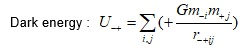The U-+ term is a positive gravitational potential energy term, a repulsive force term, and an antigravity term.

The dark energy term varies in size according to r-+. If the r-+ value changes, it can increase even as the universe expands.Fig.x. Red dot=positive mass, Black dot=negative mass, The U++, U--, and U-+ values vary depending on the arrangement of negative mass and positive mass.

In above case, the U-+ value is b) > a).

Look back at the above computer simulation results.Pair dist. is a kind of r-+ value. As the r-+ value decreases, the U-+ value increases.

Pair dist. :                   1.00  -->   0.19    -->  0.10         --> 0.01

U++(Matter) :            1,600 --> 1,607  --> 1,616     --> 1,607

U-+(Dark energy) :   4,200 --> 8,475 --> 13,232    --> 102,952

Negative masses are attracted to the galaxies, and as clustering increases, the U-+ value increases.

Other mechanisms may also exist, see the paper. P25~

Another possibility is that current mainstream astronomy has 3~4 major problems, which can lead to inaccurate results.

1) Negative energy, two kinds of the gravity source, antigravity

2) Dark energy

3) Dark matter

4) Problem of space expansion, Incomplete of long distant measurement. (1/R)=1+z

Mainstream astronomy is built on the assumption that space expands. However, this spatial expansion model may be wrong. The Friedman equation strongly suggests the possibility that the space expansion model has some problem.

In my opinion, spatial expansion model may be wrong.

1. Friedmann equation suggests that the spatial expansion model is wrong.

Consider a ΛCDM model called standard universe model!

We estimate that space(or vacuum) will have a constant energy density ρ. According to this, if “the expansion of the universe” is a phenomenon caused by “expansion of the space”, it is said that the space of the same size should have the same energy density even if the time changes.

Thus, if the expansion of the universe is a phenomenon resulting from the expansion of space, the same size distance must always have the same recession velocity, even though time goes by. Perhaps this is what we usually deduce about space, regardless of the ΛCDM model.

Now, let's consider the Friedmann equations!

H^2=(a'/a)^2=(8πG/3)ρ + (other terms)

The Friedmann equation suggests that the Hubble constant(or variable) is a function of the energy density ρ. It also suggests that the Hubble constant depends not only on the energy density of the space(or vacuum) but also on the energy density of the matter. Therefore, as the universe expands, the energy density ρ decreases and the Hubble constant also changes (the Hubble constant decrease).

That is, even though the distance is the same, it suggests that the recession velocity will change when the time changes.

The Friedmann equation suggests an ideally different result from the spatial expansion model. Friedmann equation suggests that the spatial expansion model is wrong.

At present, the long distance is estimated by the relational expression of the redshift and the scale factor from the spatial expansion model or assumption.

1/R = 1+z

If the spatial expansion model is not correct, the measurement values of long distance are all corrected.

The expansion of space is not an observation. What we observe is redshift, and spatial expansion is a hypothesis for redshift. The spatial expansion model is dangerous because it is not verified in the laboratory.

2. The Spatial Expansion Model does not acquire its present status by comparing the precision with the Doppler Shift Model. The model acquired the present status by the wrong stereotypes. (Hubble's observation isn't valid in place where is not the center of expansion and there is no repulsion on the cosmic scale.)

1)In the early 20th century it was a time when it was hard to think of repulsion or anti-gravity on a cosmic scale.

Since it was difficult to think of repulsion on a universal scale, we needed a virtual idea like the expansion of space. Although there is Einstein's case, he officially gave up the declaration. Also, it seems that there was an advantage that model analysis was easy. The same advantages as assuming cosmological principles.

But now we are living in a time when we have to consider repulsion in the cosmic scale.

2)Wrong stereotype that Hubble's observation isn't valid in place where is not center of expansion

It is assumed that interpretation issues of observation results above applied most in physicists and astronomers introducing expansion of space. When observed from Earth, it is observed that all galaxies recede from Earth and the recession velocity also follow all relations of V = HR.

To explain this, if position of the Earth is the center of expansion, namely if position of the Earth is the center of universe, this issue can be simply solved but it can be clearly known that Earth isn't the center of the universe from the observation of the universe until now. It is because Earth isn't the center of the solar system, but is clear to be just a planet and that the solar system isn't the center of the galactic system either.

Therefore, physicists and astronomers had to find a way to explain this and as this couldn't be explained by dynamics, a new concept that “space expands” was introduced. To explain more specifically, it is assumed that the stereotype that Hubble's observation isn't valid in place where is not center of expansion had influence.

By the way, if we actually analyze it, we can get the Hubble law even if it is not the center of the universe.

Let's just look at the logical structure.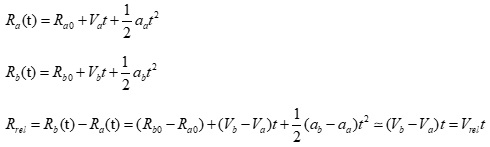In the beginning of the universe, two galaxies were very close.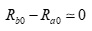Assuming that the difference in acceleration is zero,

a) Assuming that all galaxies have the same acceleration, or

b) Assuming that the acceleration term is small, or

c) Assuming that the effects of deceleration and acceleration expansion cancel each other out, and consequently this term is close to zero. ). The result of mainstream astronomy is known to be close to 3).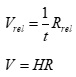If acceleration term is small, we get a Hubble’s law.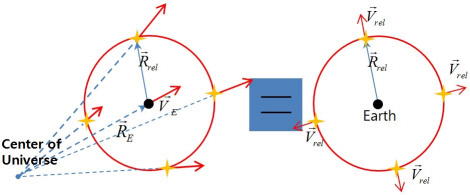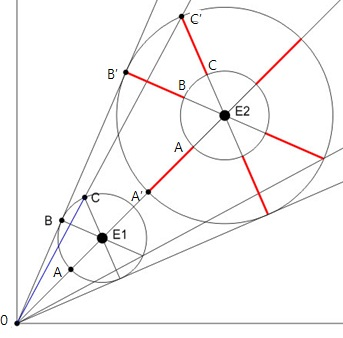Figure x. So that all of the red lines are the same size, the ones where the tangent is the line of expansion too. It's important also that the lengths are proportional, so for example OB/OB' is equal to OC/OC' and OA/OA' since velocity from the center needs to be proportional to distance. A->A', B->B', C->C', We get the Hubble law. The second figure is drawn by user Iggy.

Hubble's law isn't a matter only explained by special condition such as “center of the universe” or a new concept that we haven't experienced such as “expansion of space".

Hubble’s law can be a result of dynamics valid in almost all areas when acceleration term is small in the universe.

In addition, there was a problem that the accuracy of the Hubble constant was low in the 20th century.

3) Wrong champion match

The space expansion model wins by competing with the Tired Light Model, then occupies the standard model position. In my think, it was the wrong championship game. Its competitor had to be a Doppler Shift Model.

We know that both models give similar values for z ≤2. Since the difference between the two models is smaller than the variability of the Hubble constant, before the 2000s, there was no ability to distinguish between the two models.

In the condition z ≤2, Both models show a difference of less than 5%.

This is smaller than the fluctuation(50~100) of the Hubble constant.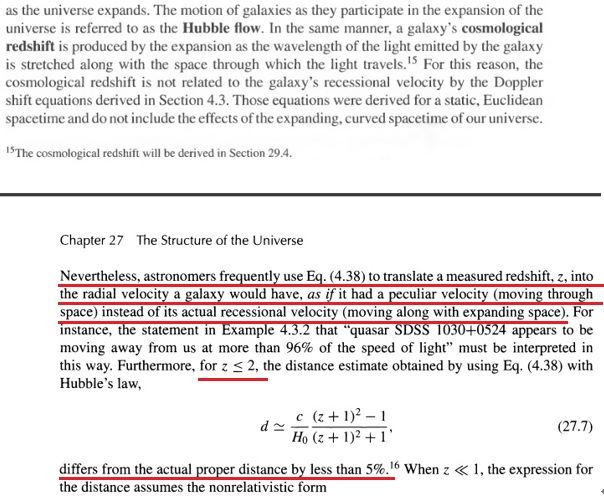For Z=2, the comoving radial distance, which goes into Hubble's law, is 5273.0 Mpc or 17.198 Gly.

Until the early 2000s, we did not have the technology to accurately measure the Hubble constant.(50~100 --> 74,72,68, again 74? km/s/Mpc)

That is, until the early 2000s, we did not have the ability to compare the accuracy of the spatial expansion model and the Doppler shift model. The Spatial Expansion Model does not acquire its present status by comparing the precision with the Doppler Shift Model.

They can’t explain why space expands and why it has a specific velocity. We did not observe the “expansion of space" directly, but observed redshift only.

If the assumption “space expands" is wrong, the relation equation of the redshift and scale factor become wrong.

This is a very important issue and needs verification.

There are currently three problems(Dark energy, Dark matter, Spatial expansion model = Imperfection of long distance measurement.) that can greatly shake the results of mainstream astronomy.

Edited by icarus2

##### Share on other sites
2 hours ago, icarus2 said:

They can’t explain why space expands and why it has a specific velocity. We did not observe the “expansion of space" directly, but observed redshift only.

If the assumption “space expands" is wrong, the relation equation of the redshift and scale factor become wrong.

This is a very important issue and needs verification.

And of course the CMBR, and to a lesser extent the particle model, Hydrogen/Helium abundance and  galactic distribution.

Quote

There are currently three problems(Dark energy, Dark matter, Spatial expansion model = Imperfection of long distance measurement.) that can greatly shake the results of mainstream astronomy.

Perhaps...we;ll just need to wait and see.

##### Share on other sites
4 hours ago, icarus2 said:

They can’t explain why space expands and why it has a specific velocity. We did not observe the “expansion of space" directly, but observed redshift only.

So much FAIL in one sentence. You do an amazing job, with every post, of demonstrating that you don't have a clue.

Expansion was predicted (ie explained) years before it was first observed.

Expansion does not have a "velocity", however the rate of expansion is determined by the density of mass-energy in the universe.

We have far more evidence than just redshift data.

##### Share on other sites

Far Far more evidence for expansion than just redshift. Thermodynamics and the changes in Blackbody temperature of the CMB as well as the CMB itself for two other pieces of evidence.

Icarus its obvious you never took the time to understand the FLRW metric in the first place and it is apparent you simply copied the equations you refer to without understanding any meaning behind those equations. Specifically those involving the FLRW metric system. For example the FLRW metric has no Centre or preferred location of the universe.

This lack of understanding is apparent in your usage of Newtons gravitational force equation which describes specifically a central potential force that you have been misapplying in every stage of your model. Your simulated images are a clear sign of this misapplication and as you can see you have a higher density towards the centre.

However measurements in Cosmology does not show the same distribution. The CMB for example is incredibly uniform in mass distribution and all measurements of our universe matches a uniform mass distribution. The below images look nothing like what we actually observe in Cosmology.....

I can pretty much quarantee you will follow the precise same pattern and ignore any comments or questions that compete against your model. As you have yet to address any of those questions. For example the repeated question.

How can you have a positive energy density but a negative mass ? Will you ever address this key and important question ?

Hubble's law is simple but it does not calculate proper distances. It calculates the recessive velocity which isn't a true velocity but an apparent (illusional) velocity based on separation distance.

"The greater the distance the greater the recessive velocity." $V_{resessive}=H_0D$ That is all Hubbles law states. Note this is not the true velocity of any galaxy but simply represents the apparent velocity. Every galaxy has roughly the same velocity as the Milky way. No galaxy has a true velocity greater than c. The greater than c recessive velocity past Hubble horizon isn't a true inertial velocity.

Your model cannot calculate the proper distance to any object in space while the FLRW metric can. It cannot calculate how temperature and pressure evolve not predict expansion contraction rates. In other words it cannot predict everything that the FLRW metric can. Here is a clear demonstration of the terms that the FLRW metric can calculate.

${\small\begin{array}{|c|c|c|c|c|c|}\hline T_{Ho} (Gy) & T_{H\infty} (Gy) & S_{eq} & H_{0} & \Omega_\Lambda & \Omega_m\\ \hline 14.4&17.3&3400&67.9&0.693&0.307\\ \hline \end{array}}$ ${\small\begin{array}{|r|r|r|r|r|r|r|r|r|r|r|r|r|r|r|r|} \hline a=1/S&S&z&T (Gy)&R (Gly)&D_{now} (Gly)&D_{then}(Gly)&D_{hor}(Gly)&D_{par}(Gly)&V_{gen}/c&V_{now}/c&V_{then}/c&H/Ho \\ \hline 0.001&1090.000&1089.000&0.000373&0.000628&45.331596&0.041589&0.056714&0.000856&21.023&3.148&66.182&22915.263\\ \hline 0.001&739.062&738.062&0.000713&0.001172&45.031283&0.060930&0.083238&0.001668&16.621&3.127&51.977&12283.974\\ \hline 0.002&501.112&500.112&0.001342&0.002163&44.653685&0.089109&0.122010&0.003214&13.287&3.101&41.203&6658.325\\ \hline 0.003&339.773&338.773&0.002496&0.003956&44.183524&0.130038&0.178562&0.006124&10.712&3.068&32.869&3639.803\\ \hline 0.004&230.379&229.379&0.004601&0.007192&43.602350&0.189264&0.260828&0.011554&8.691&3.028&26.316&2002.235\\ \hline 0.006&156.206&155.206&0.008416&0.013015&42.887747&0.274559&0.380106&0.021616&7.083&2.978&21.095&1106.404\\ \hline 0.009&105.913&104.913&0.015309&0.023478&42.012463&0.396668&0.552333&0.040144&5.791&2.918&16.895&613.344\\ \hline 0.014&71.813&70.813&0.027726&0.042257&40.943206&0.570134&0.799715&0.074095&4.745&2.843&13.492&340.773\\ \hline 0.021&48.692&47.692&0.050056&0.075939&39.639382&0.814081&1.152677&0.136056&3.894&2.753&10.720&189.626\\ \hline 0.030&33.015&32.015&0.090158&0.136321&38.051665&1.152552&1.651928&0.248752&3.200&2.642&8.455&105.633\\ \hline 0.045&22.386&21.386&0.162117&0.244527&36.119894&1.613538&2.350040&0.453165&2.631&2.508&6.599&58.889\\ \hline 0.066&15.178&14.178&0.291145&0.438335&33.771262&2.224979&3.311204&0.823085&2.164&2.345&5.076&32.852\\ \hline 0.097&10.291&9.291&0.522342&0.785104&30.917756&3.004225&4.606237&1.491191&1.782&2.147&3.827&18.342\\ \hline 0.143&6.978&5.978&0.936102&1.403692&27.454972&3.934517&6.297233&2.695518&1.470&1.907&2.803&10.259\\ \hline 0.211&4.731&3.731&1.674119&2.496871&23.266389&4.917511&8.402147&4.860753&1.219&1.616&1.969&5.767\\ \hline 0.312&3.208&2.208&2.977691&4.373615&18.247534&5.688090&10.827382&8.733318&1.026&1.267&1.301&3.292\\ \hline 0.460&2.175&1.175&5.215425&7.334123&12.397762&5.699693&13.279345&15.569626&0.903&0.861&0.777&1.963\\ \hline 0.678&1.475&0.475&8.789420&11.115281&6.042158&4.096813&15.275613&27.272101&0.878&0.420&0.369&1.296\\ \hline 1.000&1.000&0.000&13.787206&14.399932&0.000000&0.000000&16.472274&46.278944&1.000&0.000&0.000&1.000\\ \hline 1.468&0.681&-0.319&19.704190&16.201608&4.910267&7.207286&16.992292&75.113899&1.305&0.341&0.445&0.889\\ \hline 2.154&0.464&-0.536&26.084608&16.928765&8.515267&18.345587&17.174536&118.018864&1.833&0.591&1.084&0.851\\ \hline 3.162&0.316&-0.684&32.638034&17.180008&11.040250&34.912335&17.224075&181.212698&2.651&0.767&2.032&0.838\\ \hline 4.642&0.215&-0.785&39.249711&17.261713&12.776339&59.302512&17.261713&274.042078&3.872&0.887&3.435&0.834\\ \hline 6.813&0.147&-0.853&45.880114&17.287747&13.962589&95.126009&17.287747&410.320588&5.675&0.970&5.503&0.833\\ \hline 10.000&0.100&-0.900&52.516301&17.296130&14.771503&147.715032&17.296130&610.357404&8.326&1.026&8.540&0.833\\ \hline 14.678&0.068&-0.932&59.154549&17.298683&15.322788&224.907769&17.298683&903.973904&12.218&1.064&13.001&0.832\\ \hline 21.544&0.046&-0.954&65.793394&17.299445&15.698407&338.211934&17.299445&1334.944709&17.933&1.090&19.550&0.832\\ \hline 31.623&0.032&-0.968&72.432255&17.299812&15.954315&504.519738&17.299812&1967.523376&26.322&1.108&29.163&0.832\\ \hline 46.416&0.022&-0.978&79.071348&17.299828&16.128669&748.626510&17.299828&2896.022178&38.636&1.120&43.274&0.832\\ \hline 68.129&0.015&-0.985&85.710288&17.299959&16.247453&1106.926069&17.299959&4258.871858&56.709&1.128&63.984&0.832\\ \hline 100.000&0.010&-0.990&92.349407&17.299900&16.328381&1632.838131&17.299900&6259.261851&83.237&1.134&94.384&0.832\\ \hline \end{array}}$

As you can see this is far more testable and predictive not to mention practical than anything your model shows. I can take any redshift value and I can give you the proper distance both when the signal was first received and the proper distance today.
I can also tell you what temperature will correspond to any redshift value.

This chart matches observational evidence and matches the graphs of the FLRW metric in for example Lineweaver and Davies.

So please start addressing the questions being posed and respond to the question.
How can you have negative mass with a positive energy density ?

You will note the above also predicts well into the future in terms of how the universe will continue to expand.

Proper distance for example with curvature term k=o is $d_p=\int^\sigma_0\frac{d\sigma}{\sqrt{1-k\sigma}}$  where $\sigma$ is a dimensionless commoving coordinate. K is specifically the Gaussian curvature term given by $K=\frac{3}{\pi} \lim_{s \to 0}\frac{2\pi-C}{s^3}$

Here is more info on Gaussian curvature you will note I used a different notation this is the notation Matt Roose uses in Introductory to Cosmology.

Edited by Mordred

##### Share on other sites
13 hours ago, Mordred said:

I can pretty much quarantee you will follow the precise same pattern and ignore any comments or questions that compete against your model.

Unfortunately, he/she has been doing this for as long as I have been on (various different) science forums - and that’s quite a few years now. It’s just the same things being reposted over and over again, without any indication whatsoever that he/she is receptive to what is being said in response.

Your post is excellent Mordred, but I think it will - in this case - fall on deaf ears.

##### Share on other sites
On 2018. 6. 10. at 12:59 AM, Mordred said:

How do you get negative mass from something with a positive energy density such as the cosmological constant.

On 2018. 6. 23. at 2:22 PM, Mordred said:

This is a positive energy density, (all energy density values are positive....) explain how this gives negative mass ?

We do not know what the origin of dark matter and dark energy is. There are hundreds of models because it is not confirmed. Opinions differ among researchers.

Therefore, we must distinguish among the knowledge we have, what is observed and what is the hypothesis or model for it. And we must think that any explanation of a problem can be an assertion only within their model.

What we observe in the universe is the redshift. Since then, much of the content is hypothetical and model. Cosmological constant, vacuum energy, Quintessence, F(R) theory, MOND, WIMP, Axion, Sterile neutrino... It is a hypothesis and a model.

What we have to explain is the redshift or the accelerating expansion, not the positive cosmological constant.

So, what equation can explain the acceleration expansion of the universe?

It is a second Friedmann equation and an acceleration equation.

From the second Friedmann equation or acceleration equation,Since ρ is the energy density in the acceleration equation, the pressure term P also has a dimension of energy density(c=1). Acceleration equation inevitably require negative (gravitational) mass density. In the mainstream astronomy, without negative mass density, it is impossible to create acceleration expansion.

However, depending on individual hypotheses and models, there are different ways to produce negative mass density.

1. Negative mass model : Existence with negative inertial mass and negative gravitational mass

In the negative mass model, negative mass density is essential and no manipulation is required.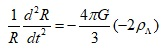The right side becomes a positive value, so accelerating expansion.

From the observance of the HSS team and SCP team in 1998, they gained the negative mass density, using field equations which do not have the cosmological constant.

The first findings were as follows:

HSS(The High-z Supernova Search) team : Λ=0, Ω_m= - 0.38(±0.22) : negative mass density

P14 and Table 8

SCP(Supernova Cosmology Project) team : Λ=0, Ω_m=-0.4(±0.1) : negative mass density

The negative mass meets that condition (negative gravitational mass density) from the beginning.

2.Mainstream model : cosmological constant or vacuum energy

The cosmological constant or vacuum energy is defined as having a positive (inertial) energy density, positive inertial mass. This alone does not produce acceleration expansion. They introduce the negative pressure and put the negative energy density into the negative pressure term.

The logic or trick they create is to introduce a presence with a positive inertia mass, acting on negative pressure.

Their logic is this.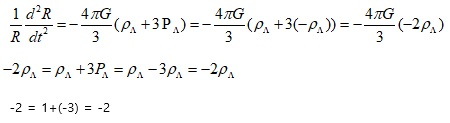Mainstream cannot accelerate expansion without negative (gravitational) mass density. What we need is -2.

It can have -2 from the beginning. = negative mass model

1 + (-3) can also make -2.                = mainstream

By the way, if we think about it carefully, we can see that "- 2" is avoided, but "- 3" problem remains.

======

How do you get negative mass from something with a positive energy density such as the cosmological constant.

======

Therefore, this question is a question that should be asked to mainstream physics and astronomy.

Look at the cosmological constant or vacuum energy

1) Fine tuning problem : 10^120 times

2) Cosmological Constant Coincident Problem

3) Energy conservation problem

The opinions are divided. Some people think that it's a problem, and others think that it's not a problem.

4) Equivalent principle of inertial mass and gravitational mass is in violation

Gravitation and Spacetime – by Hans C. Ohanian and Remo Ruffini

======

Expressed in another way, the inertial mass density for the cosmological term is positive, but the gravitational mass density is negative.

======

By plotting the claims of the mainstream,

Inertial mass density = + 1ρ (=Λ/8πG), gravitational mass density = - 2ρ (=- Λ/4πG) : not only different in sign, but also different in size. It is contrary to the equivalent principle of inertial mass and gravity mass, which is the basis of general relativity theory.

By introducing negative pressure, people think that acceleration expansion is explained and the problem is solved ~, not at all.

Many people are not aware of the serious problems of objects with negative pressure with positive energy density.

5) Sign of the negative pressureIn the acceleration equation, the source of the pressure term 3P is the kinetic energy, and 3P has the idea of an equivalent mass density corresponding to the kinetic energy of the particle.

So, assuming that the pressure P term has a negative energy density is same assuming that it has negative kinetic energy. In order to have negative kinetic energy, it must have negative inertial mass. But, because the mainstream assumed a positive inertial mass, this is a big problem. It is a logical contradiction.

6) Size of the negative pressure

In the ideal gas state equation, we obtain,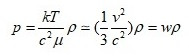Pressure P is related to kinetic energy. For a radiation with the greatest velocity, P = (1/3)ρ.

In the case of mater, v<<c, w=0

In the case of radiation, v=c, w=1/3

By the way, how can we physically create three times w larger than light? How can 3(1/3)?

How do we get the size 1 of |w|?

If we assume an object with superluminal speed square root(3)*c , can we explain it?

Well, we can avoid this problem by assuming that cosmological constant or vacuum energy does not apply to the results(ideal gas state eq.) obtained through mainstream physics. However, ideal gas state equation applies to “massless to infinite mass(There is no upper limit)” particles, and the velocity ranges from “0 to c” are all included. The coverage of this equation includes a range that is difficult to ignore.

We think that the dark energy problem has been solved by introducing the existence of positive inertial mass density and negative pressure.

However, if you seriously consider the physical presence of P = -ρ = -3(ρ/3), you will find that there is a serious problem. The problem has not been solved, but the problem has turned into another.

The cockroach came out as food, and no one ate it. The chef put cockroaches in chocolate bars and most people do not feel any resistance it. The cockroach is still there~

the inertial mass density for the cosmological term is positive, but the gravitational mass density is negative.

For someone, cosmological constants and vacuum energy will look beautiful.

However, hide the information about the person who made it, think that you saw the claim.

It can change its size by 10^120 times, and the energy conservation law chews and can adjust its own inertial mass and gravitational mass freely. It is supposed to be moving faster than light.

What I want to say is that the game is not over yet. Dark energy is not determined to be cosmological constant or vacuum energy.

Although the cosmological constant or vacuum energy is at the forefront, there are many serious problems. And it is not the situation that other runners have given up. Therefore, we must distinguish among the knowledge we have, what is observed and what is the hypothesis or model for it. And we must think that any explanation of a problem can be an assertion only within their model.

##### Share on other sites
On 02/06/2018 at 10:38 AM, studiot said:

Please explain what you mean by 'electrostatic self energy'?

On 23/06/2018 at 2:44 PM, studiot said:

This is reminiscent of the caculation of Madelung constants.

Have you heard of these?

You should be able to derive similar overall constants for your hypothesis.

Now I have done you the courtesy of offering constructive comments and asking a couple of simple questions that can support simple answers rather than long screeds that seem to be prefaced by such get out clauses as " We assume that".........

Now please provide a simple direct answer to a simple direct question.

Still no answers to either of my simple questions

Reported.

##### Share on other sites
4 hours ago, icarus2 said:

What I want to say is that the game is not over yet.

!

Moderator Note

Yes, it is.

You have failed to adequately support your arguments in this speculative thread, and have ignored repeated requests for clarity and answers to simple questions. You've had 3 pages to persuade the membership about the validity of your idea, and now seem to just be preaching and not listening. That's not a discussion, that's a blog.

This thread is closed. Do NOT bring this topic up again.